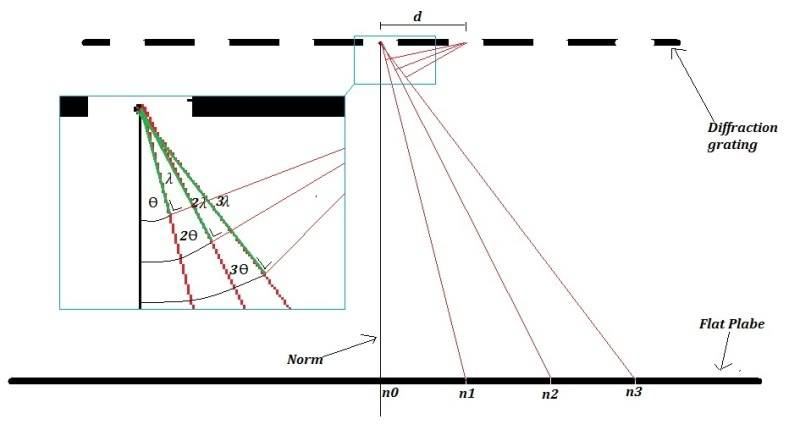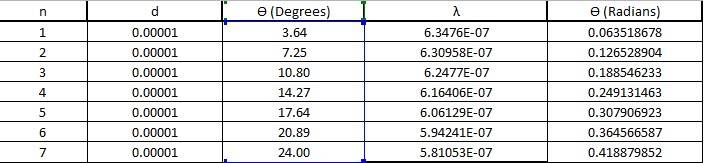# Calculating the wavelength of a laser? (Diffraction grating)

• Modrisco
The distances between the bright spots are not exactly equal, but I used the same equation to calculate the other two angles.f

#### Modrisco

Hi, I'm doing a experiment/report on this topic, I'm trying to get a better understanding of the equation "nλ = dsinθ"

ALL length measurements are in meters for simplicity's sake.

n = bright spot number from the central bright spot (n0)

d = distance between slits

The angle "θ" and distance between slits "d" are known in this experiment.

I drew a diagram to represent how "I think" it works! basically what would happen when monochromatic light would do as it passes through diffraction grating!
The image is zoomed in very close!
I know that this equation is derived from trigonometry, but I be correct in what I am saying in the diagram?Another question I have:

Is the "nλ = dsinθ" flawed? what I noticed was, if you look at the results below, the wavelength seems to be inverse proportional to "n"...is this possibly slight errors I did during the experiment? or is it because the further the waves spread the less wave length it will have?Sorry if this seems silly, I've been trying to crack it for hours...if someone can advise me I would be grateful, but please keep your answer dumbed down as much as possible for me to understand!:P

Last edited:
How did you get the theta values? How far was the screen from the grating? What were the distances of the bright spots from the central ones?

ehild

I'll explain the method 1st:

-Laser was pointed at a metre stick (standing sideways)
-Diffraction grating was placed in front of the laser
-The distance "D" from the metre stick to diffraction grating was measured.
-distance between the bright spots (x) shining onto the meter stick was measured

Using the values:

D = 0.566 m
x = 0.036 m

I was able to calculate the theta value:

tan θ = x/D
tan θ = (0.036)/(0.566)
tan θ = 0.064
θ = tan^-1(0.064)
θ = 3.63 degrees

Last edited:
I see how you got the data for the first spot but what about the others?
If xn is the distance of the n-th spot from the centre, the n-th theta is θn = tan-1(xn/D).
The spots are not quite equidistant.

ehild

So you're saying...that the distance between "n0" and "n1" is not the quite equal to the distance between "n1" and "n2"?So basically the distances between each bright spot are not exactly equal?
Would this explain why the wavelength is slowly declining in length as you go up in "n" in the table I put up?

Last edited:
To get the other 2 values of theta, for n2
I just assumed the distance from n0 to n2 was twice the distance of n0 to n1

So I used the same equation you put in:

θn = tan-1(xn/D).

But doubled the value for x for n2, and tripled value for n3

That's how calculated the other 2 angles...

I'm assuming that's not the way to go about it? since the distances between the bright spots are not equidistant

You should have read the positions of all spots on the metre stick. From all x values, you would get a theta and a lambda. And those lambda values would scatter around the real one.

ehild